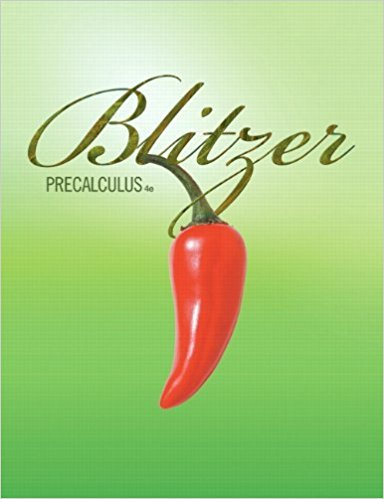×
×

# Solutions for Chapter 7.6: Linear Programming## Full solutions for Precalculus | 4th Edition

ISBN: 9780321559845Solutions for Chapter 7.6: Linear Programming

Solutions for Chapter 7.6
4 5 0 343 Reviews
11
4
##### ISBN: 9780321559845

This expansive textbook survival guide covers the following chapters and their solutions. This textbook survival guide was created for the textbook: Precalculus, edition: 4. Since 39 problems in chapter 7.6: Linear Programming have been answered, more than 71243 students have viewed full step-by-step solutions from this chapter. Chapter 7.6: Linear Programming includes 39 full step-by-step solutions. Precalculus was written by and is associated to the ISBN: 9780321559845.

Key Calculus Terms and definitions covered in this textbook
• Arccosine function

See Inverse cosine function.

• Blind experiment

An experiment in which subjects do not know if they have been given an active treatment or a placebo

• Cofunction identity

An identity that relates the sine, secant, or tangent to the cosine, cosecant, or cotangent, respectively

• Compounded annually

See Compounded k times per year.

• Constraints

See Linear programming problem.

• Control

The principle of experimental design that makes it possible to rule out other factors when making inferences about a particular explanatory variable

• Degree

Unit of measurement (represented by the symbol ) for angles or arcs, equal to 1/360 of a complete revolution

• Gaussian elimination

A method of solving a system of n linear equations in n unknowns.

• Hyperboloid of revolution

A surface generated by rotating a hyperbola about its transverse axis, p. 607.

• Instantaneous velocity

The instantaneous rate of change of a position function with respect to time, p. 737.

• Left-hand limit of f at x a

The limit of ƒ as x approaches a from the left.

• Linear regression

A procedure for finding the straight line that is the best fit for the data

• Mathematical induction

A process for proving that a statement is true for all natural numbers n by showing that it is true for n = 1 (the anchor) and that, if it is true for n = k, then it must be true for n = k + 1 (the inductive step)

• Mathematical model

A mathematical structure that approximates phenomena for the purpose of studying or predicting their behavior

• Multiplication principle of probability

If A and B are independent events, then P(A and B) = P(A) # P(B). If Adepends on B, then P(A and B) = P(A|B) # P(B)

• Outcomes

The various possible results of an experiment.

• Rectangular coordinate system

See Cartesian coordinate system.

• Reflection through the origin

x, y and (-x,-y) are reflections of each other through the origin.

• Semiminor axis

The distance from the center of an ellipse to a point on the ellipse along a line perpendicular to the major axis.

• Unbounded interval

An interval that extends to -? or ? (or both).

×